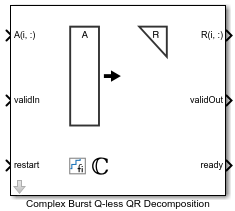# Complex Burst Q-less QR Decomposition

Q-less QR decomposition for complex-valued matrices

• Library:
• Fixed-Point Designer / Matrices and Linear Algebra / Matrix Factorizations

•## Description

The Complex Burst Q-less QR Decomposition block uses QR decomposition to compute the economy size upper-triangular R factor of the QR decomposition A = QR, where A is a complex-valued matrix, without computing Q. The solution to A'Ax = B is x = R\R'\b.

### Creation

`fixed.getQlessQRDecompositionModel(A,B)` generates a template model containing a Complex Burst Q-less QR Decomposition block for complex-valued input matrices A and B.

## Ports

### Input

expand all

Rows of complex matrix `A`, specified as a vector. A is a m-by-n matrix where m ≥ 2 and n ≥ 2. If A is a fixed-point data type, A must be signed and use binary-point scaling. Slope-bias representation is not supported for fixed-point data types.

Data Types: `single` | `double` | `fixed point`
Complex Number Support: Yes

Whether inputs are valid, specified as a Boolean scalar. This control signal indicates when the data at the A(i,:) input port is valid. When this value is 1 (`true`) and the value at ready is 1 (`true`), the block captures the values at the A(i,:) input port. When this value is 0 (`false`), the block ignores the input samples.

Data Types: `Boolean`

Whether to clear internal states, specified as a Boolean scalar. When this value is 1 (`true`), the block stops the current calculation and clears all internal states. When this value is 0 (`false`) and the validIn value is 1 (`true`), the block begins a new subframe.

Data Types: `Boolean`

### Output

expand all

Rows of the economy size QR decomposition matrix R, returned as a scalar or vector. R is an upper-triangular matrix. The output at R(i,:) has the same data type as the input at A(i,:).

Data Types: `single` | `double` | `fixed point`

Whether the output data is valid, specified as a Boolean scalar. This control signal indicates when the data at output port R(i,:) is valid. When this value is 1 (`true`), the block has successfully computed the matrix R. When this value is 0 (`false`), the output data is not valid.

Data Types: `Boolean`

Whether the block is ready, returned as a Boolean scalar. This control signal indicates when the block is ready for new input data. When this value is `1` (`true`) and the validIn value is `1` (`true`), the block accepts input data in the next time step. When this value is `0` (`false`), the block ignores input data in the next time step.

Data Types: `Boolean`

## Parameters

expand all

Number of rows in input matrix A, specified as a positive integer-valued scalar.

#### Programmatic Use

 Block Parameter: `m` Type: character vector Values: positive integer-valued scalar Default: `4`

Number of columns in input matrix A, specified as a positive integer-valued scalar.

#### Programmatic Use

 Block Parameter: `n` Type: character vector Values: positive integer-valued scalar Default: `4`

## Extended Capabilities

### Functions

Introduced in R2020a

## SupportGet trial now